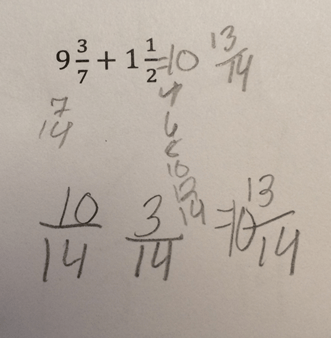Categories

# 3/7 = 10/14 and 1/2 = 3/14Nathan sends along a really lovely fraction mistake.

If I’ve got this right, the kid added 3 and 7 to get the numerator, and added 1 and 2 to get the numerator? This is a way of thinking about fractions that’s new to me. Can anyone offer a better theory or some helpful context for this kids’ thinking?

## 6 replies on “3/7 = 10/14 and 1/2 = 3/14”

My first thought was that he knew he could not add 3/7 and 1/2 without first finding a common denominator, which he did: 14. He added (in the case of 3/7, 7 to the numerator and denominator to get 10/14 and in the case of 1/2, 12 to the numerator and denominator to get 13/14, which he then wrote down because it was the last fraction he had in his mind. But that wouldn’t explain where the 3/14 came from, so you’re probably right. What’s also not helpful is that the answer is correct.LSquaredsays:

Sadly, considering that the answer is correct, my best guess was that the student saw the answer on the paper of the student next to him or her and then backfilled to find some plausible looking numbers that it might have come from. Which means that the main thing it shows is that this student has little or no idea where common denominator equivalent fractions come from.Gabesays:

I agree with LSquared that there’s a reasonable chance the student started with the answer and tried to come up with it. That being said, my guess is that the student has been doing a lot of work converting mixed numbers to fractions and recalls part of that process involves adding something involving the denominator to the numerator and keeping the denominator.Niels Johnsensays:

Just found this wonderful site – I’m a teachers’ educator from Denmark.
We don’t have to assume that the student meant 3/7=10/14 and 1/2=3/14.
Here’s another explanation: This student multiplies 2 x 7 to get 14, the common denominator. I see no other plausible explanation for the counting in steps – 7, 14 and 2,4,6,8,12,14. So I actually think this student understands common denominators very well .
The student does not explain or document his or her thinking about the numerators. However, 10/14 and 3/14 might be explained otherwise than the assumption in the headline. Assume the student succesfully converted 3/7 = 6/14 and 1/2=7/14 and now adds the numerators to get 13/14 – and for some reason splits to tens and ones.finkelitissays:

Here’s my theory: the student finds the common denominator by counting up by sevens and twos, respectively. Then the students needs to convert the numerators, but doesn’t remember exactly how to do it. So the kid adds the denominator of each fraction to the numerator (7+3 and 1+2) and gets 10 and 3 as the numerators.stephensays:

this didnt fucking work i got a B minus these bitches